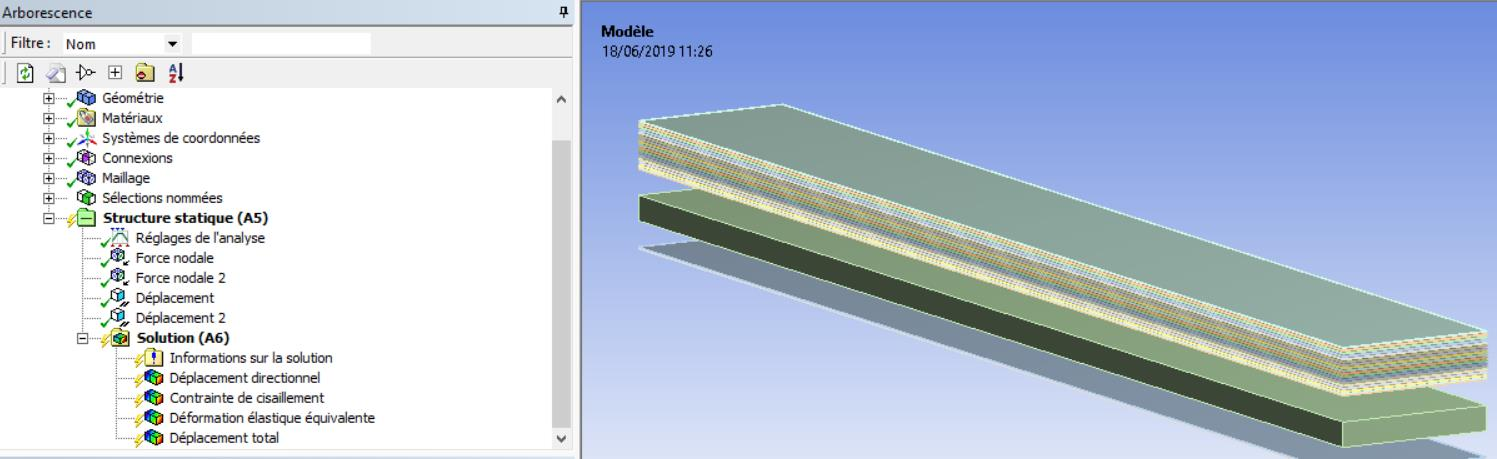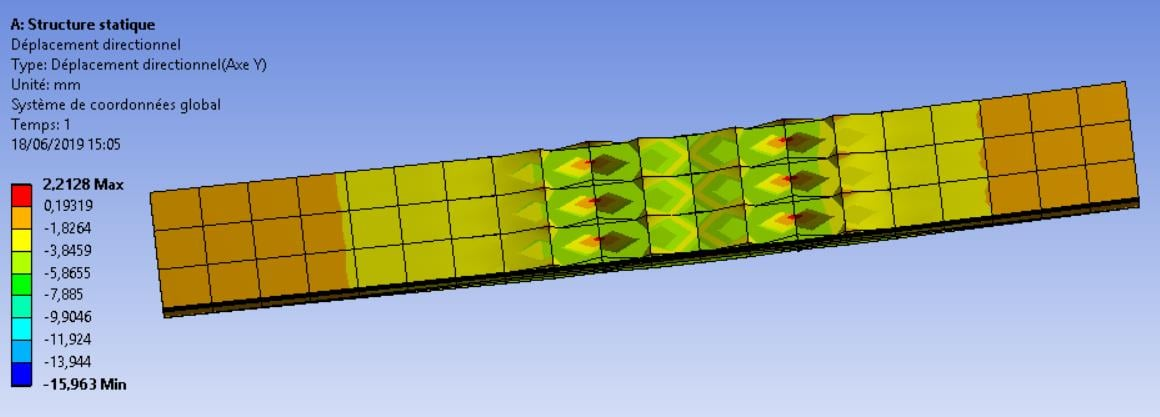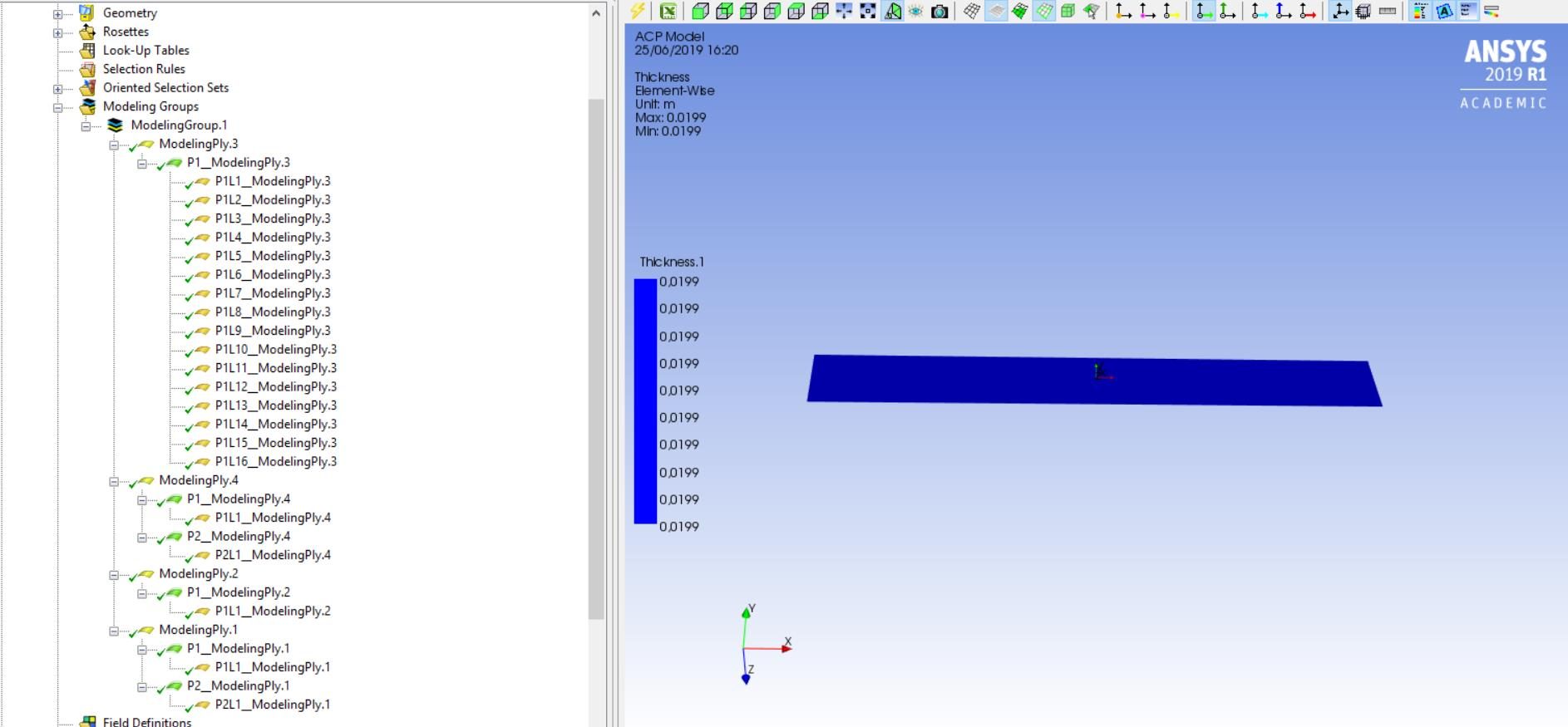## General Mechanical

•Jonathan974
Subscriber

Hello everyone,

I would like to realize an analysis of a beam's response under  a 4 point bending test in order to get the curve : Force VS Deflection on a node. It would be a non linear curve I guess.

The beam is composed by 4 materials as shows the picture (2FaceSheets-1Core-2FaceSheets-16Composite)Unfortunately when I solve the project after have applying my boundaries conditions (I've applied 2 nodal forces and fixed translations on edges of the beam), I get this result :Maybe this is due to connections between each layers ( I've automatically created contacts area ).

Would it be more coherent to work with ACP system components instead of a static structure ? Using  2D orthotropic propertie for each materials is that correct to get a non linear solution ?

Project is joined,

J.

•peteroznewman
Subscriber

1) Don't use Nodal Force to push down on this beam. The elements are quadratic, which means pushing each node down with an equal force does not result in a uniform pressure along the line of nodes.  You can use a nodal displacement instead, but it is better to slice the geometry to get an edge where it is needed.

2) You don't have small enough elements, though you may be limited by the Student license limit of 32,000 nodes.

3) Use Symmetry to solve 1/4 of the problem by slicing the model in half about two plane through the center.

4) Don't use Contact to connect the layers. Use Shared Topology

5) Use SOLSH190 for bending thin layers of material, but you must define the Mesh Control as Sweep and select a Source face to define the through thickness direction and then set Solid Shell as the type of element.

6) Use more than one element through the thickness of the sandwich core layer, which is thicker than all the thin layers.

7) Under Analysis Settings, Change to Auto Time Stepping and set the Initial and Minimum Substeps to 10. Turn on Large Deflection.

Even with all those changes, it is still possible that local buckling of the face sheet is the failure mode and that can be an accurate result, but you will need much smaller elements to see that clearly.

•Jonathan974
Subscriber

Hi,

The core is actually a honeycomb panel, do I need to use specified elements to deal with ?

I let you know results asap,

Thanks ! J.

•Jonathan974
Subscriber

Hello, I update this topic with a new model,

So I re worked it with ACP, problem is solved but results are not coherent. When I turn off the large deflection option I've got a linear solution (F=k*x) but I would obtain a non linear solution just as F=A*(1-exp(-B*x)) and I am obtaining a kind of simply exponential model which goes to infinity with the large deflection option. So I guess this is not a model problem's (this is a simple 4 points test bending)

Maybe I need to edit a limit before break of something like that ? Or maybe I need to precise non-linearity of materials I use into Engineering Data ?

Here is my modeling plies :Thank you !